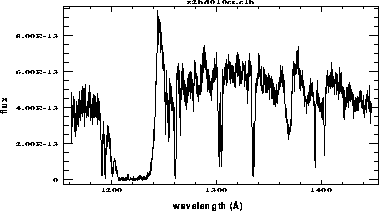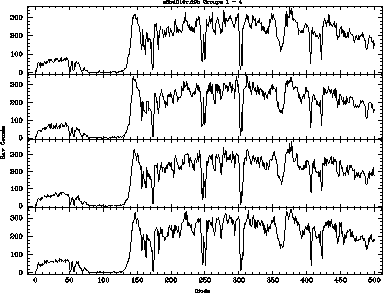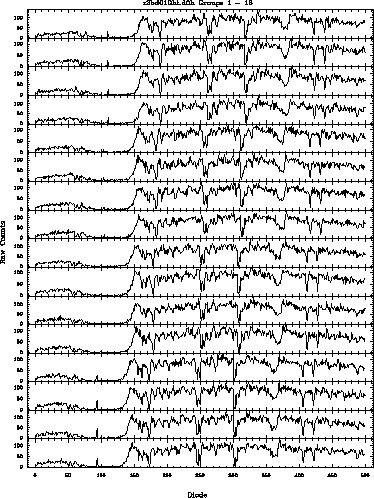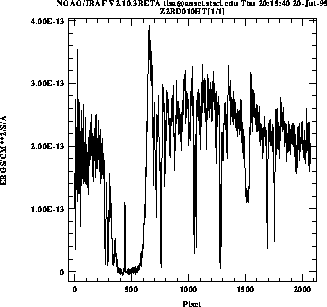[Top] [Prev] [Next] [Bottom]

# 35.6 Assessing GHRS Science Data

## 35.6.1 Examining the ACCUMs

Here we return to our examination of program 5297. There are many, many data files for this program, but to start you would probably like to look at the reduced spectra, and then go back to understand how they got the way they are and how the calibrations might be improved.

The reduced wavelength and flux files have suffixes of .c0h and .c1h (for a complete explanation of file suffixes, see Table 35.1). GHRS observations have separate files for wavelengths because they are on a non-linear scale; i.e., there is a distinct wavelength associated with each output data point, and those wavelengths are not necessarily evenly spaced. For a quick look, we need to use fwplot, a task in stsdas. The fwplot task will display flux versus wavelength and it can find the right wavelength file automatically (unless you changed its name), so we use the following to examine the first group of a repeat observation containing R136a2:

```cl>fwplot z2bd010ct.c1h

```
This produces the plot shown in Figure 35.12. Plots of the other spectra can be obtained by changing the rootname. fwplot can also plot error bars and the like, which we will explain in a moment.

#### Figure 35.12: Flux vs. Wavelength for R136a2Now that we have seen the spectrum, let's understand better why it looks the way it does. First let's look at counts versus pixel to get a sense of the quality of the observations. Since there are three repeats in this observation, we will look at all four of the corresponding groups of data. We must create a listing of the file names with the group numbers attached, so we use grlist and redirect the output to a file. Then we stack the spectra using sgraph.

```cl> grlist z2bd010ct.d0h - > lis
cl> sgraph @lis st+

```
Note how few counts we have at the short-wavelength (left) end (Figure 35.14). We can see the effect of this in the reduced spectrum. If we use fwplot to plot the a small part of the reduced spectrum with its errors (Figure 35.13) we get essentially the same information in a more compact form, and we can see that the errors are the statistical ones calculated from raw counts.

#### Figure 35.14: Raw Counts vs. Diode Number for Four Spectra in z2bd010cThe reduced spectrum in Figure 35.13 has a wavelength scale that is the default from the pipeline reduction system. There are two ways to improve on this, either by using a wavelength calibration exposure or by using a SPYBAL. For example, we can take the SPYBAL z2bd010bt and process it with waveoff. The SPYBAL exposure is shown in Figure 35.15, and the net result is that we compute a wavelength offset of 0.708 Å. See the help file for waveoff for examples of how to use it.

## 35.6.2 Putting FP-SPLITs Back Together

Specifying FP-SPLIT=FOUR (or any value except "NO") on the exposure logsheet resulted in small motions of the grating carrousel between individual subexposures. This moved the source spectrum slightly on the diode array, making it possible to distinguish fixed-pattern noise from true spectrum features and to correct for that pattern.

The most sophisticated use of FP-SPLITs involved iteration to determine a function representing the fixed-pattern noise. This could be augmented by obtaining spectra with larger discrete motions of the grating as well.1 Here we describe the simplest use of FP-SPLITs for achieving more modest gains in signal-to-noise. This involves simply realigning the individual subexposures so that spectrum features line up, and then adding up the spectra.

#### Figure 35.16: Raw FP-SPLIT Observations for R136a5Note that there are four groups of four (due to STEPPATT=3), with a shift in wavelength after every fourth spectrum (due to the FP-SPLIT setting). The result of shifting and adding all the subexposures for R136a5 is shown in Figure 35.17 using sgraph to show that there are more pixels in the combined spectrum than in the output of the pipeline software.

#### Figure 35.17: Summed Spectrum for R136a5[Top] [Prev] [Next] [Bottom]

1 See the discussion by Cardelli et al,. 1993, ApJ., 402, L17; Cardelli and Ebbets 1994, in Calibrating Hubble Space Telescope, J.C. Blades and A.J. Osmer eds., (Baltimore: STScI), 322.

stevens@stsci.edu
Copyright © 1997, Association of Universities for Research in Astronomy. All rights reserved. Last updated: 01/14/98 15:45:39